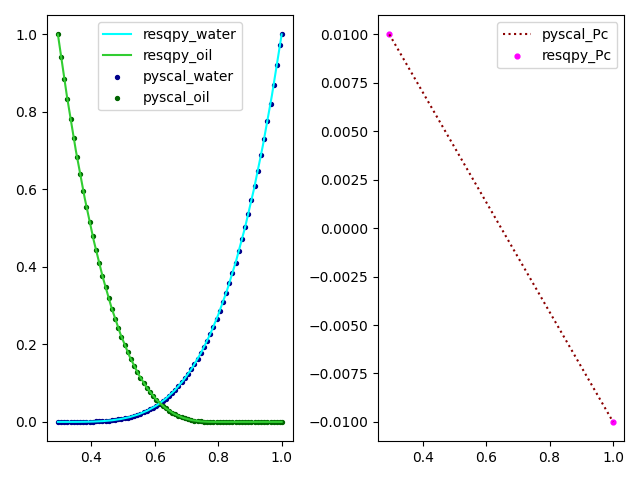# Working with the RelPerm class and equinor/pyscal¶

This tutorial describes two workflows that allow us to pass relative permeability and capillary pressure data between the resqpy library and the equinor\pyscal library.

We will be moving water-oil relperm data between resqpy’s `resqpy.olio.relperm.RelPerm` class and pyscal’s `pyscal.WaterOil` class.

Please note that similar workflows can be used for moving gas-oil data to/from the `pyscal.GasOil` class.

## Importing the relperm and wateroil modules¶

In this tutorial we will be moving water-oil relperm data between the `RelPerm` object’s `resqpy.olio.relperm.RelPerm.dataframe()` method and the `WaterOil` object’s `pyscal.WaterOil.table` attribute.

```from resqpy.olio.relperm import RelPerm
from pyscal import WaterOil
# import a plotting library for visual inspection of the data
import matplotlib.pyplot as plt
# import resqpy model module to interact with a resqpy model
import resqpy.model as rm
```

## resqpy RelPerm.dataframe() to pyscal WaterOil.table¶

Initialize an instance of a `RelPerm` object that is stored in a resqpy `Model` instance. This can be done using the uuid of the existing `Grid2dRepresentation` object that acts as support for the dataframe of relperm data.

```model = rm.Model('/path/to/my_file.epc')
relperm_wo = RelPerm(model = model, uuid = uuid)
```

The dataframe of water-oil relperm data is then accessed using the `resqpy.olio.relperm.RelPerm.dataframe()` method.

```relperm_wo_df = relperm_wo_obj.dataframe()
```

To initialize a pyscal `WaterOil` object, we first need to define a water saturation end-point that is compatible with the `RelPerm` dataframe being inputted. In this case, we can define swl, which will be the first water saturation in the generated `WaterOil` table, and set it equal to the minimum water saturation value in the `RelPerm` dataframe.

```swl = relperm_wo_df.min()['Sw']
pyscal_wo = WaterOil(swl = swl)
```

The pyscal `pyscal.WaterOil.add_fromtable()` method generates a relperm table by interpolating relative permeability and capillary pressure data from the inputted dataframe.

```pyscal_wo.add_fromtable(dframe = relperm_wo_df, swcolname = 'Sw', krwcolname='Krw', krowcolname='Kro', pccolname='Pc')
pyscal_wo_df = pyscal_wo.table
```

We’ll generate some plots to visually compare the inputted `RelPerm` data to the `WaterOil` data.

```fig, (ax1, ax2) = plt.subplots(nrows = 1, ncols = 2)
ax1.plot(relperm_wo_df['Sw'], relperm_wo_df['Krw'], label = 'resqpy_water', c = 'cyan')
ax1.plot(relperm_wo_df['Sw'], relperm_wo_df['Kro'], label = 'resqpy_oil', c = 'limegreen')
ax1.scatter(pyscal_wo_df['SW'], pyscal_wo_df['KRW'], label = 'pyscal_water', c = 'darkblue', s = 8)
ax1.scatter(pyscal_wo_df['SW'], pyscal_df['KROW'], label = 'pyscal_oil', c = 'darkgreen', s = 8)
ax1.legend( )
ax2.scatter(relperm_wo_df['Sw'], relperm_wo_df['Pc'], label = 'resqpy_Pc', c = 'magenta', s = 12)
ax2.plot(pyscal_wo_df['SW'], pyscal_wo_df['PC'], label = 'pyscal_Pc', linestyle = 'dotted', c = 'darkred' )
ax2.legend( )
fig.tight_layout()
plt.show()
```

The image below compares two sets of relperm and capillary pressure data:## pyscal WaterOil.table to resqpy RelPerm.dataframe()¶

Moving data in the opposite direction is simple, and involves reformatting the column names of the `WaterOil` table to be compatible with the `RelPerm` initialiser method. We reference the same `WaterOil` table instance, pyscal_wo_df, from the previous section.

```model = rm.Model('/path/to/my_file.epc')
all_relevant_pyscal_cols = ['SW', 'SG', 'KRW', 'KRG', 'KROW', 'KROG']
cols = sorted(list(set(pyscal_wo_df.columns).intersection(set(all_relevant_pyscal_cols))), reverse=True)
if 'PC' in pyscal_wo_df.columns:
cols.append('PC')
col_remap_dict = {k: (k.capitalize() if len(k) < 4 else k.capitalize()[0:3]) for k in cols}
pyscal_wo_df_processed = pyscal_wo_df[cols].rename(columns = col_remap_dict)
# intialize a new RelPerm object, write hdf5 and create xml for object
relperm_wo = RelPerm(model = model, df = pyscal_wo_df_processed)
relperm_wo.write_hdf5_and_create_xml()
```